# Cora Documentation – Overview of WeightSets I: $\mathbb{Z}$, $\mathbb{Q}$, $\mathbb{R}$, $\mathbb{C}$. ¶

(version 04/04/2019 - Awali 1.0.2)

Awali deals with weighted automata. The weights are taken in sets that are called internally WeightSets and that are, in a first approximation, semirings. This first document (which is cut in two parts indeed) presents the numerical semirings that are available in the current version of Awali. A next document will present more complex WeightSets , and another one how a user can define its own WeightSet and integrate it in Awali.

The list of numerical semirings that are available is given at the end of the output of the command cora help:

B, Z, Q, R, C, F2, Z-min-plus, Z-max-plus, R-max-prod, Z/<int>Z



The name are explicit. Besides for the last one which is peculiar as it depends from a parameter given by the user, the above writing is the way the semiring is denoted in the -W option.

Boolean automata have been described in the document GettingStartedWithCora. We review here, in this first part, the commands for semirings Z, Q, R and C. In a second part of Cora Documentation - Weights, we review the commands for the remaining semirings.

These documents serve the double purpose of the presentation of the available functions in Cora and the systematic test of them. Hence the redundance and the repetition of the same functions for the various semirings. Very few commands indeed are specific to certain WeightSets: determinize is valid for Boolean automata, reduce for Z- or X-automata where X is a field, are-equivalent for B-, Z- or X-automata. All other commands are valid for all WeightSets.

### Nota Bene¶

Cora is the command-line interface of Awali, hence the ! prepending each command below. We use Jupyter notebook as a presentation tool and do not recommend using Cora through Jupyter in general. Indeed, some core features of Cora have no real interface with Python/Jupyter, such as display, edit or autocompletion.

## 1. Weights in $\mathbb{Z}$¶

### 1.1 Generalities: display, support, characteristic, eval¶

There are several preloaded $\mathbb{Z}$-automata in Awali: b1, c1, d1, binary.

In :
!cora display b1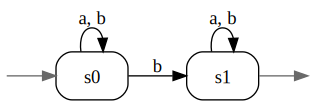As warned in the Nota Bene, the display command does not draw the figure below the cell in the notebook but opens another window on the screen.

In :
!cora display c1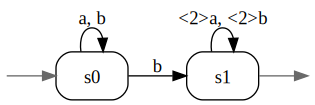It is seen on this figure that weights are written between < > (as in weighted rational expressions), and that the weight is not explicitely written when it is equal to the identity element of the weight semiring (indeed, when this identity element is written $1$).

The latter assertion can be witnessed when using Z-min-plus WeightSet (with some kind of anticipation) in relation with the support and characteristic functions. A weighted automaton can be "stripped" from its weights and sent to a Boolean automaton by the command support. Conversely, a Boolean automaton can be transformed into a weighted automaton, with any prescribed weight semiring, by the command characteristic with the adequate -W option.

In :
!cora support b1 \| -WZ-min-plus characteristic - \| display -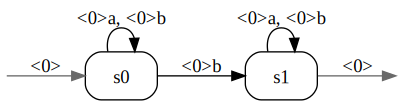### 1.2 eval¶

The automaton b1 "counts" the number of occurences of $b$'s in a word (over $A=\{a,b\}$). This can be observed with the command eval which associates with every word its coefficient in the series realised by the automaton, or with the command enumerate.

In :
!cora eval b1 'bab'

2

In :
!cora enumerate b1 3

<1> b
<1> ab
<1> ba
<2> bb
<1> aab
<1> aba
<2> abb
<1> baa
<2> bab
<2> bba
<3> bbb


The above line shows that enumerate yields the list of words with a non zero coefficient, in the radix order (the same command with awalipyyields the list in the lexicographic order).

The automaton binary is the same as c1 but for the fact that label $a$ is replaced by $0$ and label $b$ by $1$. The automaton binary associates with every word over $A_{2}=\{0,1\}$ the value it represents in base $2$.

In :
!cora eval binary '101'
!cora eval c1 'abba'

5
6


The automaton d1 " counts" the difference between the number of $a$'s and the number of $b$'s in a words over $A=\{a,b\}$.

In :
!cora eval d1 'abaab'

1


CAVEAT If the word given to the command eval is not over the alphabet of the automaton, no error is detected (no exception is raised). The word simply does not label any successful computation of the automaton and then the coefficient of the word evaluates to $0$.

In :
!cora eval d1 'abacab'

0

In :
!cora support c1 \| -WZ characteristic - \| eval - 'bab'

2


### 1.3 Constructive functions: product, quotient and reduction¶

The product, and the power, commands yield the tensor product, or power, of the automata which realise the Hadamard product of the behaviour of the automata.

In :
!cora power c1 3 \| eval - 'bab'

125


One can compute the minimal quotient or the minimal co-quotient of a weighted automaton

In :
!cora power c1 3 \| min-quotient - \| display -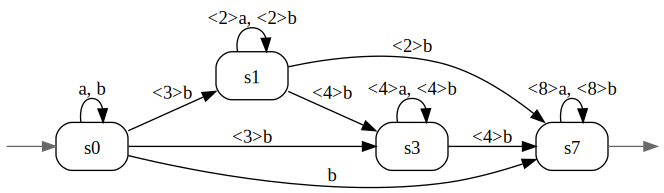In :
!cora power c1 3 \| min-coquotient - \| display -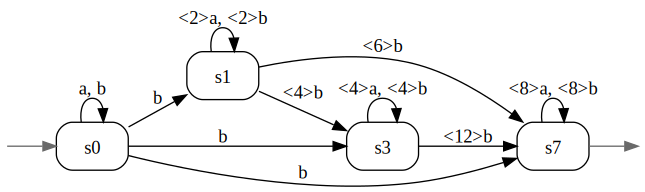A $\mathbb{Z}$-automaton can also be reduced (because $\mathbb{Z}$ is a principal ideal domain -- almost a field).

In :
!cora power c1 3 \| reduce - \| display -      # (*) #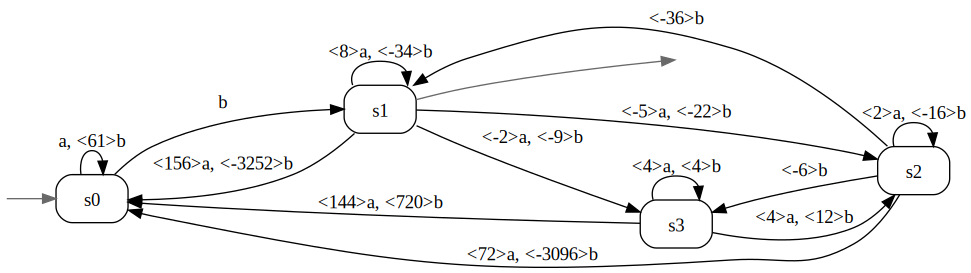It is not so obvious that this new automaton is equivalent to the two others above. And still they are and this can be checked with Awali (since reduction is computable for $\mathbb{Z}$-automata.

In :
!cora power c1 3 \| min-quotient - > c3min.json
!cora power c1 3 \| reduce - > c3Zred.json
!cora are-equivalent c3min.json c3Zred.json

true


### 1.4 Construction of $\mathbb{Z}$-automata.¶

A first way to constructing $\mathbb{Z}$-automata is to use the edit or create commands with the -WZ option. These commands are user-friendly but not presentable in a notebook. A first alternative way is to input a $\mathbb{Z}$-rational expression.

In :
!cora -WZ exp-to-aut '(a+b)*b(<2>a+<2>b)*' > c1Z.json
!cora display c1Z.json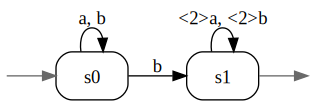This procedure gives the opportunity to study the properties of different methods for computing an automaton from an expression.

In :
!cora -WZ -Mstandard exp-to-aut '(a+b)*b(<2>a+<2>b)*' > c1Zstan.json
!cora display c1Zstan.json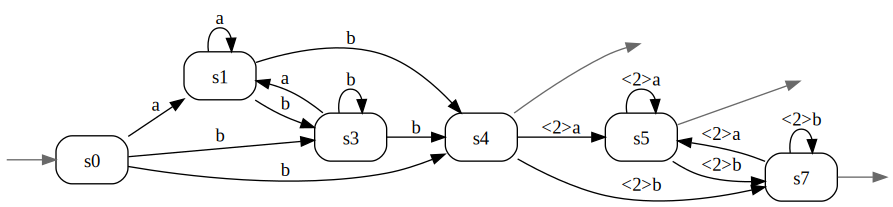In :
!cora -WZ -Mthompson exp-to-aut '(a+b)*b(<2>a+<2>b)*' > c1Zthom.json
!cora display c1Zthom.json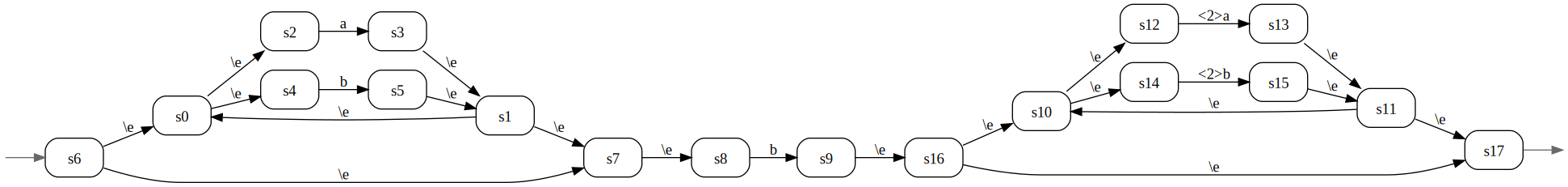In :
!cora proper c1Zthom.json \| are-isomorphic - c1Zstan.json

true

In :
!cora min-quotient c1Zstan.json \| are-isomorphic - c1Z.json

true


The last two commands illustrate the statements that the backward closure of the Thompson automaton of a rational expression is equal to the standard automaton of that expression and that the minimal quotient of the standard automaton is equal to the derived term automaton (when the latter is minimal).

The next commands show how one can lift at the level of automata the rational operations, when they are applied to standard automata.

In :
!cora -WZ -Mstandard exp-to-aut 'b' > bZ.json
!cora -Odot display bZ.json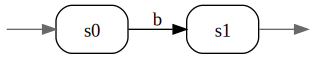In :
!cora -WZ -Mstandard exp-to-aut 'a+b' > AlphaZ.json
!cora display AlphaZ.json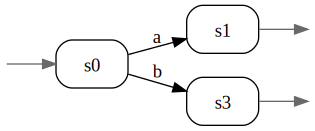In :
!cora left-mult '2' AlphaZ.json > Alpha2Z.json
!cora display Alpha2Z.json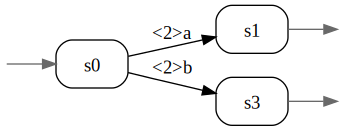In :
!cora star AlphaZ.json > AlphaeZstan.json
!cora display AlphaeZstan.json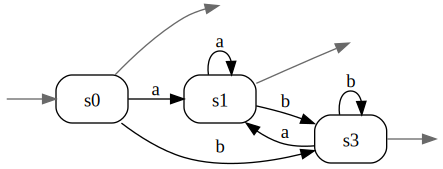In :
!cora star Alpha2Z.json > Alpha2eZstan.json
!cora display Alpha2eZstan.json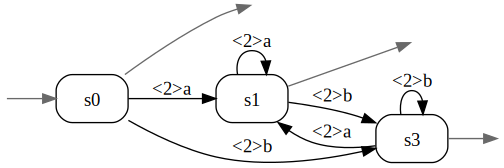In :
!cora concatenation AlphaeZstan.json bZ.json \| concatenation - Alpha2eZstan.json > c1Zbuilt.json
!cora are-isomorphic c1Zstan.json c1Zbuilt.json
!cora display c1Zbuilt.json

true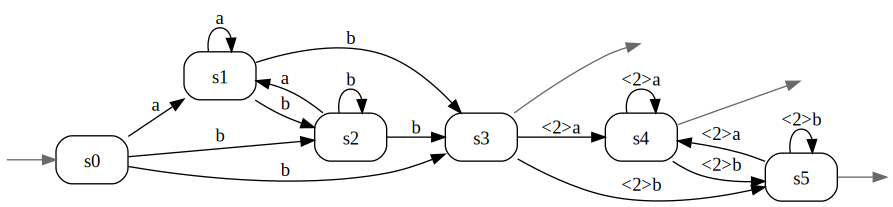The following variant may be of interest

In :
!cora -WZ -Mstandard exp-to-aut 'a+b' \| min-quotient - > AlphaZ-bis.json
!cora left-mult '2' AlphaZ-bis.json > Alpha2Z-bis.json
!cora star AlphaZ-bis.json > AlphaeZ-bis.json
!cora star Alpha2Z-bis.json > Alpha2eZ-bis.json
!cora concatenation AlphaeZ-bis.json bZ.json \| concatenation - Alpha2eZ-bis.json > c1Zbuilt-bis.json
!cora display c1Zbuilt-bis.json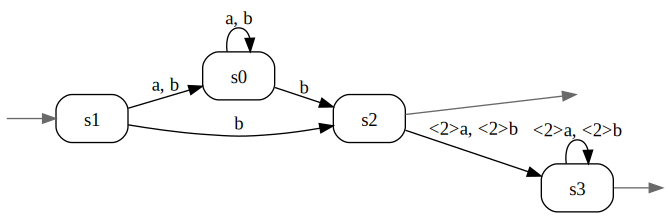To conclude this sequence, let us remark that the construction of the Thompson automaton of a rational expression -- a classic operation in the Boolean case -- may be more than problematic in the case of weighted expressions and automata: the introduction of spontaneous transitions inherent in Thompson construction may produce artefacts that yield non valid automata from valid expressions.

In :
!cora -WZ is-validE '(\e+<-1>(b*))*'
!cora -WZ exp-to-aut '(\e+<-1>(b*))*' > Z1.json
!cora is-valid Z1.json
!cora display Z1.json

true
true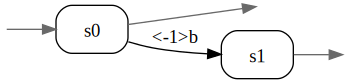In :
!cora -Mthompson -WZ exp-to-aut '(\e+<-1>(b*))*' > Z1thom.json
!cora is-valid Z1thom.json
!cora display Z1thom.json

false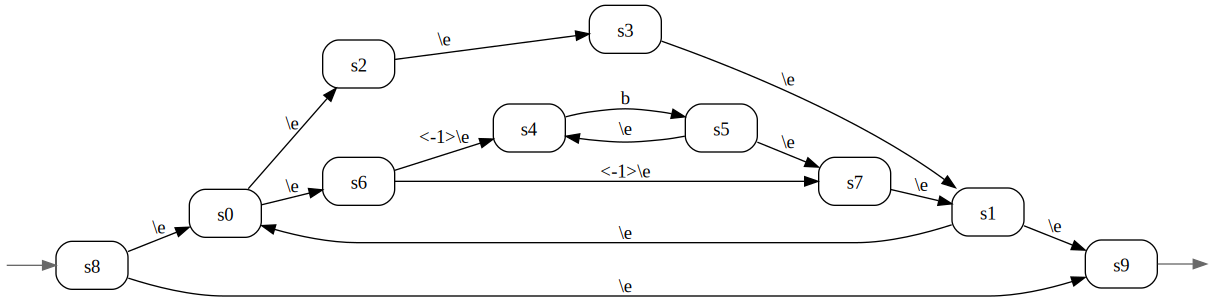### 1.5 An application¶

$\mathbb{Z}$-automata are fit to compute combinatorial results. For instance, the (generalised) binomial coefficient $\binom{abaab}{ab}$ is computed by the following sequence of commands (which is easy to write in a shell script if necessary).

In :
!cora -WZ exp-to-aut '(a+b)*' > Alphae.json
!cora -WZ exp-to-aut 'ab' > ab.json
!cora shuffle ab.json Alphae.json \| eval - 'abaab'

4


## 2. Weights in $\mathbb{Q}$¶

There is only one preloaded $\mathbb{Q}$-automaton in Awali: e1.

### 2.1 Generalities: display, eval¶

In :
!cora display e1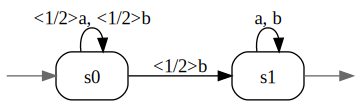The automaton e1 associates with every word of $\{a,b\}^{*}$ the value it represents in base $2$ when it is placed after the radix point. This figure shows how elements of $\mathbb{Q}$ are represented (and input): int / int. If the rational number happens to be an integer, it is then represented and input as such: int (see below the automaton c1Q.json).

In :
!cora eval e1 'abbab'

13/32


### 2.2 Construction of $\mathbb{Q}$-automata¶

As $\mathbb{Z}$-automata, $\mathbb{Q}$-automata can be built with the edit or create commands with the -WQ option and which, as above, are not presentable in a notebook. As for $\mathbb{Z}$-automata again, a possible method is to input a $\mathbb{Q}$-rational expression.

For instance, the next line generates an automaton which "the same" as c1 but with weights in $\mathbb{Q}$ rather than in $\mathbb{Z}$:

In :
!cora -WQ exp-to-aut '(a+b)*b(<2>a+<2>b)*' > c1Q.json
!cora display c1Q.json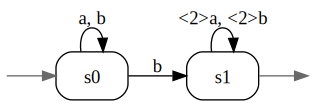The automata c1 and c1Q are "equal" but as the weight types are not the same, some functions are realised by distinct algorithms, as shown by the following line of commands.

In :
!cora power c1Q.json 3 \| reduce - \| display -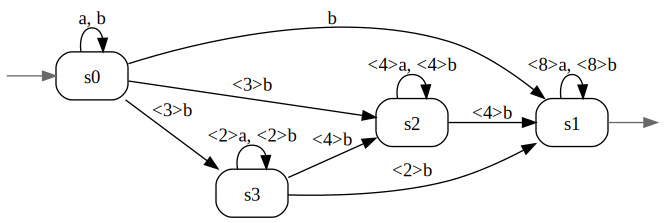This figure, when compared with the one following the instruction (*), shows that the function reduce calls distinct algorithms for $\mathbb{Z}$ and $\mathbb{Q}$-automata.

Another way of building $\mathbb{Q}$-automata is to use the characteristic function, but of course this concerns characteristic automata only, and those one can built from them.

In :
!cora support b1 \| -WQ characteristic - > b1Q.json
!cora display b1Q.json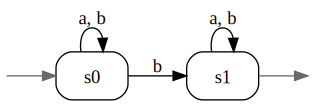Here again, the difference between the automata b1 and b1Q.json can be observed via the behaviour of the reduce function.

In :
!cora power b1 3 \| reduce - > b3red.json
!cora display b3red.json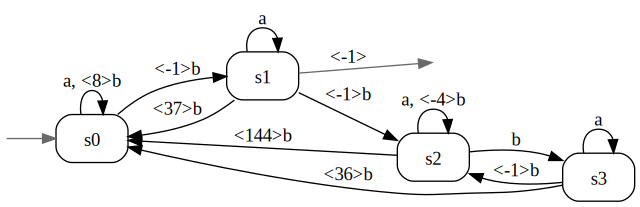In :
!cora power b1Q.json 3 \| reduce - \| display -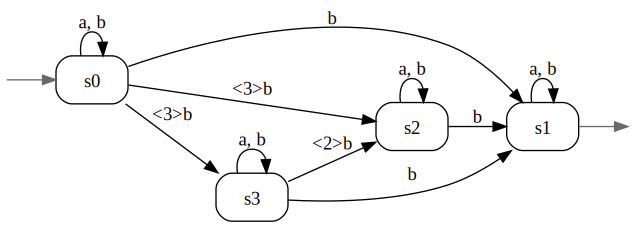Weights in $\mathbb{Q}$ also allows to deal with situations where the star of a non proper series is valid.

In :
!cora -WQ exp-to-aut '(<1/6>(a*)+<1/3>(b*))*' > Qaut1.json
!cora display Qaut1.json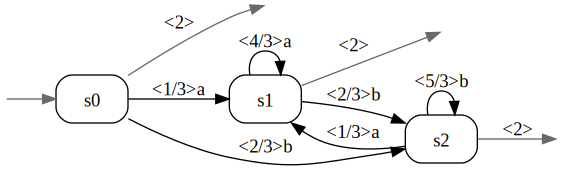### 2.3 "Promotion" of semirings¶

Another feature of Awali is the promotion of semirings. The semiring $\mathbb{Z}$, for instance, is a sub(semi)ring of $\mathbb{Q}$, its elements can then be considered as elements of $\mathbb{Q}$. It follows that operations that involve two automata -- such as the product, the union, or the shuffle -- should be allowed between a $\mathbb{Z}$-automaton and a $\mathbb{Q}$-automaton and they are indeed, and the result is a $\mathbb{Q}$-automaton.

As a consequence, any $\mathbb{Z}$-automaton can be transformed into a $\mathbb{Q}$-automaton by making the product with the characteristic ($\mathbb{Q}$)-series of the free monoid.

In :
!cora -WQ exp-to-aut '(a+b)*' > AlphaeQ.json
!cora display AlphaeQ.json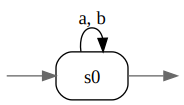In :
!cora product c1 AlphaeQ.json > c1Q-bis.json
!cora display c1Q-bis.json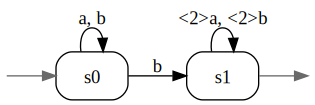In :
!cora power c1Q.json 3 \| reduce - \| display -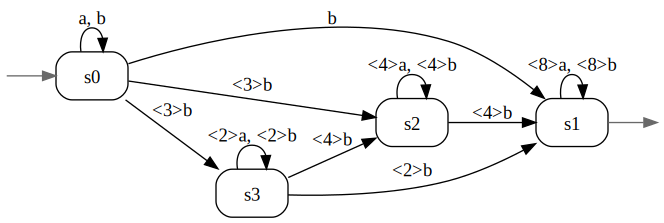## 3. Weights in $\mathbb{R}$¶

There is only one preloaded $\mathbb{R}$-automata in Awali: prob-rabin.

In :
!cora display prob-rabin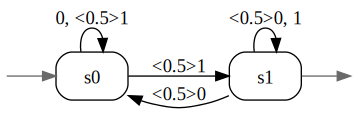In :
!cora enumerate prob-rabin 3

<0.5> 1
<0.5> 01
<0.25> 10
<0.75> 11
<0.5> 001
<0.25> 010
<0.75> 011
<0.125> 100
<0.625> 101
<0.375> 110
<0.875> 111


As for $\mathbb{Q}$-automata, there are several ways for creating $\mathbb{R}$-automata: the commands create and edit commands with the -WR option, the definitions of series with weighted rational expressions with weight in $\mathbb{R}$ and the use of the exp-to-aut command, and since $\mathbb{Q}$ is a subsemiring of $\mathbb{R}$, the product with the with the characteristic ($\mathbb{R}$)-series of the free monoid.

In :
!cora -WR exp-to-aut '(a+b)*' > AlphaeR.json
!cora display AlphaeR.json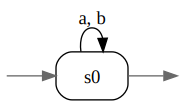In :
!cora product e1 AlphaeR.json > e1R.json
!cora display e1R.json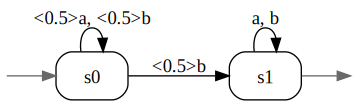In :
!cora eval e1R.json 'abbab'

0.40625


With these two commands one sees that the two automata e1 and e1R are equal but that their weights are in $\mathbb{Q}$ and $\mathbb{R}$ respectively.

Anticipating with the document on transducers, we transform the automaton prob-rabin by replacing 0 by a and 1 by b, a task that is performed by the transducer transcript.json.

In :
!cora display transcript.json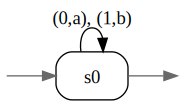In :
!cora eval-tdc prob-rabin transcript.json > prob-rabin-ab.json
!cora display prob-rabin-ab.json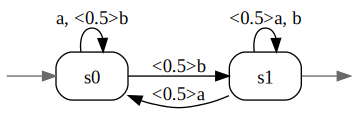The following equivalence, hinted by the command line cora enumerate prob-rabin 3 above, is not so obvious from the automata themselves.

In :
!cora transpose prob-rabin-ab.json \| are-equivalent - e1R.json

true


## 4. Weights in $\mathbb{C}$¶

There is only one preloaded $\mathbb{C}$-automaton in Awali: rotation.